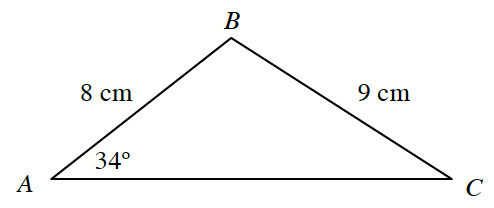### Home > PC3 > Chapter 6 > Lesson 6.2.3 > Problem6-107

6-107.Given the triangle at right, calculate $AC$.

The given information is SSA, so there could be two possible triangles.
Use the Law of Cosines to calculate both possible values of $AC$.